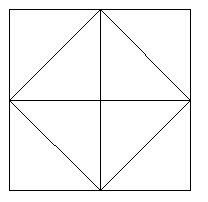# Area of squares formed by joining midpoints repeatedly in C?

CServer Side ProgrammingProgramming

#### C in Depth: The Complete C Programming Guide for Beginners

45 Lectures 4.5 hours

#### Practical C++: Learn C++ Basics Step by Step

Most Popular

50 Lectures 4.5 hours

#### Master C and Embedded C Programming- Learn as you go

66 Lectures 5.5 hours

Area of a square is equal to the product of sides of the square.

We are considering a figure in which the midpoints of sides of each square make another square. And so on until a specific number of squares.

This figure shows a square made by joining the midpoints of a square.For this figure the let the side be a,

The length of side of inner square will be

L2 = (a/2)2 + (a/2)2
L2 = a2(1/4 + 1/4) = a2(1/2) = a2/2
L = a2/ (\sqrt{2}).

Area of square2 = L2 = a2/2.

For the next square, the area of square 3 = a2/4

Lets take an example, tge

Now are we can infer from here about areas of consecutive squares,

a2, a2/2, a2/4, a2/8, …..

This is a GP with a2 being the first term of the and ½ being the common ratio.

## Example

#include <stdio.h>
#include <math.h>
int main() {
double L = 2, n = 10;
double firstTerm = L * L;
double ratio = 1 / 2.0;
double are = firstTerm * (pow(ratio, 10)) ;
printf("The area of %lfth square is %lf", n , sum);
return 0;
}

## Output

The area of 10th square is 0.003906